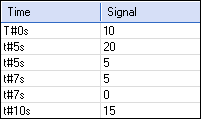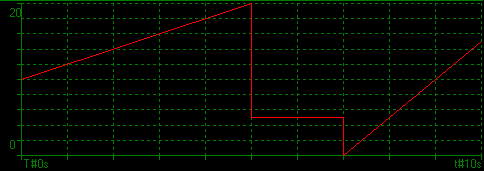# Analog signals resources

Analog signals are resources (embedded configuration data) edited with the Workbench. An analog signal is entered as a list of analog points among a time X-axis.

Signals are typically used for statically designing a set-point signal to be played at runtime using the following functions and blocks:

SigID: Get the identifier of a signal resource (to be passed to other blocks)
SigPlay: Plays a signal
SigScale: Get a point value from a signal

A signal is entered in the editor as a list of point. Each point refers to a 0-based time value (X axis) and a REAL analog value (Y axis). Below is an example:

 Points:Signal:In between two consecutive points, the signal must be understood as an analog ramp. If you need to represent a discontiguous change (vertical segment), specify the same time value (X axis) for two consecutive points. The number of points is not limited.